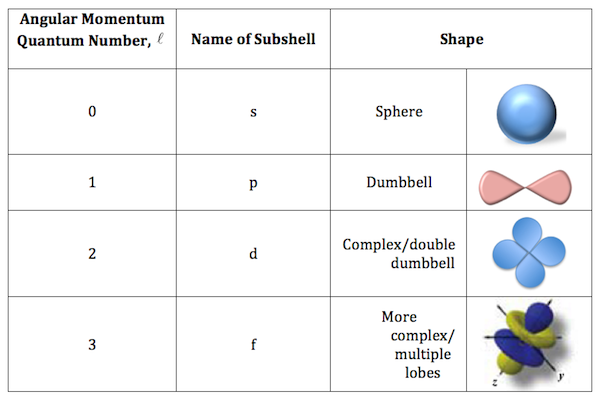## Angular Momentum Quantum Number

Moderators: Chem_Mod, Chem_Admin

Clara Cho 2K
Posts: 103
Joined: Wed Sep 18, 2019 12:18 am

### Angular Momentum Quantum Number

How does the second quantum number describe shape?

Camellia Liu 1J
Posts: 51
Joined: Sat Aug 24, 2019 12:15 am

### Re: Angular Momentum Quantum Number

The second quantum number l is the one associated with angular momentum, which determines the shape of the electron's orbital (the subshell).

The allowed values of l are: l= 0, 1, 2, ... n-1 where:
l=0 (s- orbital); l=1 (p-orbital); l=2 (d-orbital); l=3 (f-orbital)

Helen Struble 2F
Posts: 97
Joined: Sat Aug 24, 2019 12:17 am

### Re: Angular Momentum Quantum Number

The second quantum number, l, describes shape because it clues us into how many radial and angular nodes are in the subshell. With the principle quantum number, n, we can figure out how many radial and angular nodes are in a subshell. N=sum of radial and angular nodes. N = n - l - 1. So, for the 3p oribital, where n=3 and l=1, we can find that N = n - l - 1 = 3 - 1 - 1= 1 node. We can confirm this because we know that the 3p orbital is dumbbell shaped, with the angular node either being the xy plane, yz plane, or xz plane.

Eva Zhao 4I
Posts: 101
Joined: Sun Sep 29, 2019 12:16 am

### Re: Angular Momentum Quantum Number

The Angular Momentum Quantum Number (l) describes shape by corresponding with a certain sub-shell, each of which with a unique arrangement. I hope this diagram will help you better picture what the second quantum number describes; note that not all possible variations of each sub-shell are shown in the diagram.Return to “Quantum Numbers and The H-Atom”

### Who is online

Users browsing this forum: No registered users and 1 guest